# S4E3 - Molarity Calculations. How to Find Molarity of the Solution

The extent to which a solute dissolves in a solution is expressed by the solution's concentration.

## The Molarity Formula

Concentration is most often expressed as molarity (M).

Molarity (M)  =  number of moles of solute (mol)  /  liters of solution (L)

-or-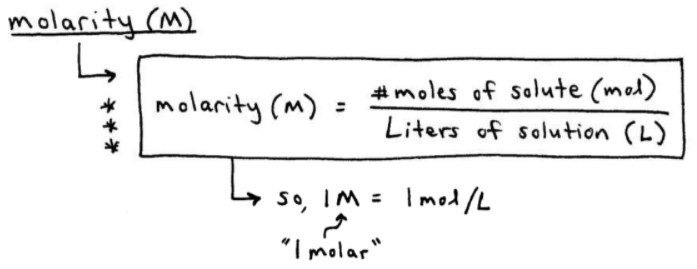The best way to understand how to use the molarity formula is to practice, practice, practice.  So let's dive in to some molarity examples now.

----------

## How to Find Molarity

ex:  Calculate the molarity of a solution made by dissolving 11.5 g of solid NaOH in enough water to make 1.50 L of solution.
_________

molarity  =  moles NaOH  /  L solution

➞  we already have the "L solution"  ➞  1.50 L

➞  but we need to calculate the "moles NaOH" before we can find the molarity...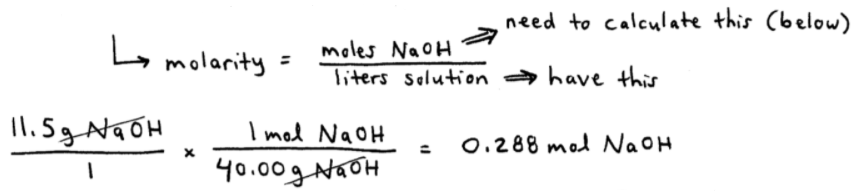Okay, so now we have both our numerator, 0.288 mol NaOH, and we always had our denominator (given), 1.50 L.  Let's divide the two and solve for molarity: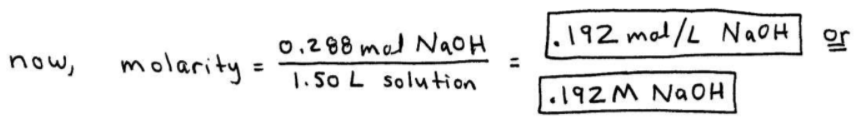----------

ex:  Give the concentration of each type of ion in a solution of 0.50 M Co(NO3)2
_________

Think mole ratios.

For each 1 mole of cobalt (II) nitrate dissolved, the solution contains 1 mole Co2+ and 2 moles NO3-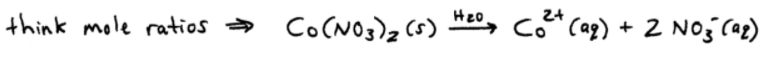So a solution that contains 0.50 M Co(NO3)2 contains:

0.50 M Co2+  and
1.0 M NO3-

----------

ex:  Calculate the number of moles of chloride ions in 1.75 L of 1.0 M ZnCl2
_________

NOTE - anytime you see [ X "L"  of  Y "M" ], you often have to multiply because  L.M  =  L.(mol/L)  =  mol

(1.75 L / 1) (1.0 mol ZnCl2 / 1L)  =  1.75 mol ZnCl2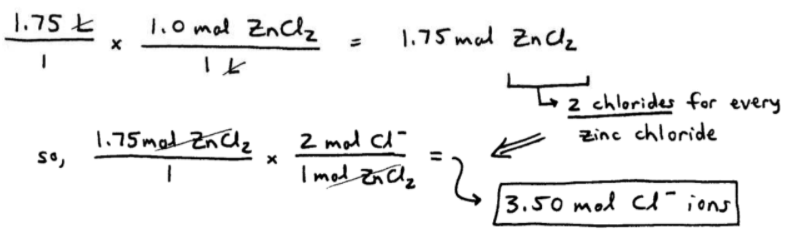----------

ex:  Blood serum is about 0.14 M NaCl.  What volume of blood contains 1.0 mg NaCl?
_________

If we convert 1.0mg NaCl to "moles NaCl," we can use the "0.14 moles / L" to get ourselves into units of liters (volume):

➞  (1.0mg NaCl / 1) (1g NaCl / 1000mg NaCl) (1mol NaCl / 58.45g NaCl)  =  1.7 x 10-5 moles NaCl

➞  (1.7 x 10-5 mol NaCl / 1) (1L solution / 0.14mol NaCl)  =  1.2 x 10-4 L blood (solution)

Here's a better look at it...----------

ex:  A chemist needs 1.00 L of an aqueous 0.200 M K2Cr2O7 solution.  How much solid K2Cr2O7 does he need to weigh out?
_________

We have [ X "L"  of  Y "M" ] ➞ L.M  =  L.(mol/L)  =  mol

➞  (1.00 L / 1) (0.200 mol K2Cr2O7 / 1L)  =  0.200 mol K2Cr2O7

➞  (0.200 mol K2Cr2O7 / 1) (294 g K2Cr2O7 / 1 mol K2Cr2O7)  =  58.8g K2Cr2O7

Here's an easy-to-follow version of the above two molarity calculations:==========

## Dilutions

People use the terms dilute and concentrated everyday to describe the contents of a liquid qualitatively.

dilute = "watered-down"
concentrated = contains "a lot" of ingredients that can be tasted

### The Dilution Equation

From a quantitative point of view, chemists can prepare "dilutions" of solutions having an actual concentration.

Usually, highly concentrated "stock solutions" are purchased by a lab, and the chemists add water to achieve the desired molarity (concentration).

The Equation:   M1V1  =  M2V2

--  M = molarity (mol / L)
--  V = volume (matching units, often mL or L)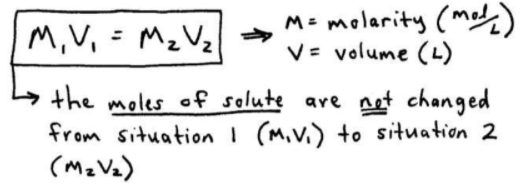As stated in the above image, the moles of solute are not changed from situation 1 (M1V1) to situation 2 (M2V2).

----------

ex:  What volume of 16 M sulfuric acid must be used to prepare 1.5 L of a 0.10 M H2SO4 solution?
_________

Well, we have:

M1 = 16 M     M2 = 0.10 M
V1 = ??             V2 = 1.5 L

➞  M1V1 = M2V2

➞  (16 M) (x L)  =  (0.10 M) (1.5 L)

➞  (16x) mol  =  0.15 mol

➞  x = 0.0094 L or 9.4 mL

Here's a better look at all that...To prepare 1.5 L of a 0.10 M H2SO4 solution, the chemist would add 9.4 mL of 16 M H2SO4 and then add enough water until the total final volume is 1.5 L.

==========

Next up in SECTION 4 - Types of Chemical Reactions and Solution Stoichiometry, we'll talk about the different Types of Chemical Reactions.